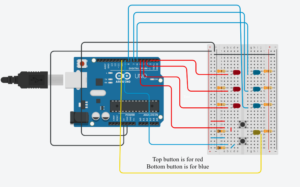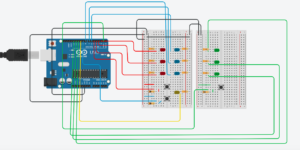# Don’t drop the light – LEDs and Buttons

#### INTRODUCTION

For this week’s assignment, I decided to make some sort of game where you have to press a button before the light reaches the bottom.
The light goes through three colored LEDs before it does reaches the bottom (which is a common yellow LED).
As I said, you have to press the corresponding colored button to “stop” the light from falling. If the light does reach the bottom/yellow LED, all the other colored LEDs will light up at once signaling you lost.
I only did this with 2 buttons, 6 LEDs (3 for each button) + an extra common LED, because including 3 buttons made it way too messy and it wouldn’t fit. However, I do include a version with 3 buttons made on an online simulator at the end.
This is what the circuit looks like for the 2-button version:#### code

I define all the pins before setup, including 2 arrays for the LED pins.
In setup(), I set the pins to OUTPUT for the LEDs, and INPUT for the buttons.
I then have two functions right before loop(). One is RED(), the other is BLUE(). They do the exact same thing, except that RED operates on the array of red LEDs, while BLUE operates on the array of blue LEDs.
Inside loop(), I just call these functions based on an if-statement.

```int rowN;
int l1 = 2;
int l2  = 3;
int l3 = 4;
int l4 = 5;
int l5  = 6;
int l6 = 7;
int b1 = 8;
int b2 = 9;
int common = 10;
int checkCommon =0; //to check if the common LED is on
int lost = 0; //to check if you lost
int pressed1 = 0; //to check if red button is pressed
int pressed2 = 0; //to check if blue button is pressed
int row1 = {l1,l2,l3,common}; //array of red LEDs
int row2 = {l4,l5,l6,common}; //array of blue LEDs
void setup() {
// put your setup code here, to run once:
for (int i= 0; i<3; i++)
{
pinMode(row1[i],OUTPUT);
}
for (int i= 0; i<3; i++)
{
pinMode(row2[i],OUTPUT);
}
pinMode(b1,INPUT);
pinMode(b2,INPUT);
pinMode(common,OUTPUT);
rowN = int(random(1,3));
}
void RED()
{
if(pressed1 == 0)
{
for (int i= 0; i<4; i++)
{
checkCommon=0;
{
pressed1 = 1;
digitalWrite(row1[i],LOW);
delay(500);
break;
}
else
{
checkCommon =1;
}
delay(200);
digitalWrite(row1[i],HIGH);
delay(100);
digitalWrite(row1[i],LOW);
if (i==3 && checkCommon ==1)
{
delay(200);
digitalWrite(common, LOW);
lost = 1;
}
}
}
if (lost == 1)
{
for (int i= 0; i<3; i++)
{
digitalWrite(row1[i],HIGH);
digitalWrite(row2[i],HIGH);
}
delay(500);
for (int i= 0; i<3; i++)
{
digitalWrite(row1[i],LOW);
digitalWrite(row2[i],LOW);
}
lost = 0;
}
if (pressed1 == 1)
{
pressed1 = 0;
}
}
void BLUE()
{
if(pressed2 ==0)
{
for (int i= 0; i<4; i++)
{
checkCommon=0;
{
pressed2 = 1;
digitalWrite(row2[i],LOW);
delay(500);
break;
}
else
{
checkCommon =1;
}
delay(200);
digitalWrite(row2[i],HIGH);
delay(100);
digitalWrite(row2[i],LOW);
if (i==3 && checkCommon ==1)
{
delay(200);
digitalWrite(common, LOW);
lost = 1;
}
}
}
if (lost == 1)
{
for (int i= 0; i<3; i++)
{
digitalWrite(row1[i],HIGH);
digitalWrite(row2[i],HIGH);
}
delay(500);
for (int i= 0; i<3; i++)
{
digitalWrite(row1[i],LOW);
digitalWrite(row2[i],LOW);
}
lost = 0;
}
if (pressed2 == 1)
{
pressed2 = 0;
}
}

void loop() {
// put your main code here, to run repeatedly:
//red case
if (rowN == 1)
{
RED();
rowN = int(random(1,3));
}
//blue case
if (rowN == 2)
{
BLUE();
rowN = int(random(1,3));
}
}```

#### Steps

First, I started out by making the LED arrays and randomly switching between them.

Then, I added the common yellow LED, and made all the other colored LEDs light up at once if the light reaches this common LED. This will mean you lost.

#### final outcome

Adding the push-buttons and their functionalities gave this final result:

#### 3 buttons version

The assignment asked for at least 3 buttons and at least 3 LEDs, but since three buttons were too hard to fit on the breadboard in my case, I made a version with three buttons on an online simulator.
This is what the circuit looks likeAnd this is what the “gameplay” looks like: